Updating search results...

# 33 Results

View
Selected filters:
• CCSS.Math.Content.6.G.A.1Educational Use
Rating
3.0 stars

In this Cyberchase video segment, the CyberSquad learns what happens to the area of a rectangle when the shape enclosed by the perimeter changes.

Subject:
Mathematics
Geometry
Material Type:
Lecture
Provider:
PBS LearningMedia
Provider Set:
PBS Learning Media: Multimedia Resources for the Classroom and Professional Development
Author:
U.S. Department of Education
WNET
07/08/2008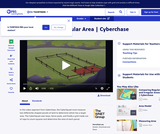Educational Use
Rating
4.0 stars

In this video segment from Cyberchase, the CyberSquad measures Judge Trudy's land claim by using tarps, fence posts and a grid made with rope.

Subject:
Mathematics
Material Type:
Lecture
Provider:
PBS LearningMedia
Provider Set:
PBS Learning Media: Multimedia Resources for the Classroom and Professional Development
Author:
U.S. Department of Education
WNET
07/07/2008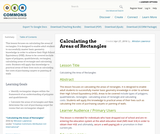Unrestricted Use
CC BY
Rating
0.0 stars

This lesson focuses on calculating the areas of rectangles. It is designed to enable adult students to successfully master basic geometry knowledge in order to achieve their High School Equivalency (HSE). Areas to be covered include types of polygons, quadrilaterals, rectangles; calculating areas of rectangle and calculating costs. Students will apply this knowledge to practical areas of their lives such as calculating the costs of purchasing carpets or painting of walls

Subject:
Mathematics
Material Type:
Interactive
Lesson Plan
Author:
Winston Lawrence
05/05/2016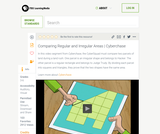Educational Use
Rating
3.0 stars

The CyberSquad proves that the area of Hacker's land is equal to the area of Judge Trudy's land in this video segment from Cyberchase.

Subject:
Mathematics
Material Type:
Lecture
Provider:
PBS LearningMedia
Provider Set:
PBS Learning Media: Multimedia Resources for the Classroom and Professional Development
Author:
U.S. Department of Education
WNET
07/08/2008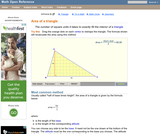Rating
0.0 stars

An interactive applet and associated web page that explain the area of a triangle. The applet shows a triangle that can be reshaped by dragging any vertex. As it changes, the area is continually recalculated using the 'half base times height' method. The triangle has a fixed square grid in its interior that can be used to visually estimate the area for later correlation with the calculated value. The calculation can be hidden while estimation is in progress. The text page has links to a similar page that uses Heron's Formula to compute the area. Applet can be enlarged to full screen size for use with a classroom projector. This resource is a component of the Math Open Reference Interactive Geometry textbook project at http://www.mathopenref.com.

Subject:
Geometry
Material Type:
Simulation
Provider:
Math Open Reference
Author:
John Page
02/16/2011Only Sharing Permitted
CC BY-NC-ND
Rating
4.5 stars

This lesson unit is intended to help teachers assess how well students are able to visualize two-dimensional cross-sections of representations of three-dimensional objects. In particular, the lesson will help you identify and help students who have difficulties recognizing and drawing two-dimensional cross-sections at different points along a plane of a representation of a three-dimensional object.

Subject:
Geometry
Material Type:
Assessment
Lesson Plan
Provider:
Shell Center for Mathematical Education
Provider Set:
Mathematics Assessment Project (MAP)
04/26/2013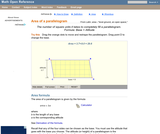Rating
0.0 stars

A web page and interactive applet showing the ways to calculate the area of a parallelogram. The user can drag the vertices of the parallelogram and the other points change automatically to ensure it remains a parallelogram. A grid inside the shape allows students to estimate the area visually, then check against the actual computed area, which is continuously recomputed and displayed. The text on the page gives three different ways to calculate the area with a formula for each. The applet uses one of the methods to compute the area in real time, so it changes as the rhombus is reshaped with the mouse. A companion page is http://www.mathopenref.com/parallelogram.html showing the definition and properties of a parallelogram Applet can be enlarged to full screen size for use with a classroom projector. This resource is a component of the Math Open Reference Interactive Geometry textbook project at http://www.mathopenref.com.

Subject:
Geometry
Material Type:
Simulation
Provider:
Math Open Reference
Author:
John Page
02/16/2011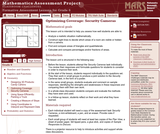Only Sharing Permitted
CC BY-NC-ND
Rating
0.0 stars

This lesson unit is intended to help sixth grade teachers assess how well students are able to: Analyze a realistic situation mathematically; construct sight lines to decide which areas of a room are visible or hidden from a camera; find and compare areas of triangles and quadrilaterals; and calculate and compare percentages and/or fractions of areas.

Subject:
Geometry
Ratios and Proportions
Material Type:
Assessment
Lesson Plan
Provider:
Shell Center for Mathematical Education
Provider Set:
Mathematics Assessment Project (MAP)
04/26/2013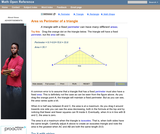Rating
4.0 stars

An interactive applet and associated web page that show the relationship between the perimeter and area of a triangle. It shows that a triangle with a constant perimeter does NOT have a constant area. The applet has a triangle with one vertex draggable and a constant perimeter. As you drag the vertex, it is clear that the area varies, even though the perimeter is constant. Optionally, you can see the path traced by the dragged vertex and see that it forms an ellipse. A link takes you to a page where this effect is exploited to construct an ellipse with string and pins. The applet can be enlarged to full screen size for use with a classroom projector. This resource is a component of the Math Open Reference Interactive Geometry textbook project at http://www.mathopenref.com.

Subject:
Geometry
Material Type:
Simulation
Provider:
Math Open Reference
Author:
John Page
02/16/2011Rating
0.0 stars

Surface Area and Volume

Type of Unit: Conceptual

Prior Knowledge

Students should be able to:

Identify rectangles, parallelograms, trapezoids, and triangles and their bases and heights.
Identify cubes, rectangular prisms, and pyramids and their faces, edges, and vertices.
Understand that area of a 2-D figure is a measure of the figure's surface and that it is measured in square units.
Understand volume of a 3-D figure is a measure of the space the figure occupies and is measured in cubic units.

Lesson Flow

The unit begins with an exploratory lesson about the volumes of containers. Then in Lessons 2–5, students investigate areas of 2-D figures. To find the area of a parallelogram, students consider how it can be rearranged to form a rectangle. To find the area of a trapezoid, students think about how two copies of the trapezoid can be put together to form a parallelogram. To find the area of a triangle, students consider how two copies of the triangle can be put together to form a parallelogram. By sketching and analyzing several parallelograms, trapezoids, and triangles, students develop area formulas for these figures. Students then find areas of composite figures by decomposing them into familiar figures. In the last lesson on area, students estimate the area of an irregular figure by overlaying it with a grid. In Lesson 6, the focus shifts to 3-D figures. Students build rectangular prisms from unit cubes and develop a formula for finding the volume of any rectangular prism. In Lesson 7, students analyze and create nets for prisms. In Lesson 8, students compare a cube to a square pyramid with the same base and height as the cube. They consider the number of faces, edges, and vertices, as well as the surface area and volume. In Lesson 9, students use their knowledge of volume, area, and linear measurements to solve a packing problem.

Subject:
Mathematics
Geometry
Material Type:
Unit of Study
Provider:
PearsonUnrestricted Use
CC BY
Rating
0.0 stars

Lesson plans on finding area of parallelograms, triangles, trapezoids, and non-regular polygons.

Subject:
Mathematics
Material Type:
Homework/Assignment
Lesson Plan
Author:
Amy Whitchurch
01/28/2016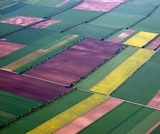Conditional Remix & Share Permitted
CC BY-NC
Rating
0.0 stars

Lesson OverviewStudents use what they know about finding the areas of basic figures to find areas of composite figures.Key ConceptsA composite figure is a figure that can be divided into two or more basic figures.The area of a composite figure can be found by dividing it into basic figures whose areas can be calculated easily.For some figures, the area can also be found by surrounding the figure with a basic figure, creating other basic figures “between” the original figure and the surrounding figure. The area of the original figure can then be found by subtracting the basic figure.Goals and Learning ObjectivesFind the area of composite figures by decomposing and composing them into more basic figures.

Subject:
Geometry
Material Type:
Lesson Plan
Provider:
Pearson
09/21/2015Only Sharing Permitted
CC BY-NC-ND
Rating
4.0 stars

This lesson unit is intended to help you assess how well students are able to: Perform arithmetic operations, including those involving whole-number exponents, recognizing and applying the conventional order of operations; Write and evaluate numerical expressions from diagrammatic representations and be able to identify equivalent expressions; apply the distributive and commutative properties appropriately; and use the method for finding areas of compound rectangles.

Subject:
Geometry
Material Type:
Assessment
Lesson Plan
Provider:
Shell Center for Mathematical Education
Provider Set:
Mathematics Assessment Project (MAP)
04/26/2013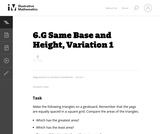Unrestricted Use
CC BY
Rating
0.0 stars

This is the first version of a task asking students to find the areas of triangles that have the same base and height, and is the most concrete.

Subject:
Mathematics
Geometry
Material Type:
Activity/Lab
Provider:
Illustrative Mathematics
Provider Set:
Illustrative Mathematics
Author:
Illustrative Mathematics
05/01/2012Conditional Remix & Share Permitted
CC BY-NC
Rating
0.0 stars

Subject:
Mathematics
Material Type:
Full Course
Provider:
Pearson
10/06/2016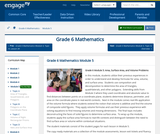Conditional Remix & Share Permitted
CC BY-NC-SA
Rating
0.0 stars

In this module, students utilize their previous experiences in order to understand and develop formulas for area, volume, and surface area.  Students use composition and decomposition to determine the area of triangles, quadrilaterals, and other polygons.  Extending skills from Module 3 where they used coordinates and absolute value to find distances between points on a coordinate plane, students determine distance, perimeter, and area on the coordinate plane in real-world contexts.  Next in the module comes real-life application of the volume formula where students extend the notion that volume is additive and find the volume of composite solid figures.  They apply volume formulas and use their previous experience with solving equations to find missing volumes and missing dimensions.  The final topic includes deconstructing the faces of solid figures to determine surface area.  To wrap up the module, students apply the surface area formula to real-life contexts and distinguish between the need to find surface area or volume within contextual situations.

Subject:
Geometry
Material Type:
Module
Provider:
New York State Education Department
Provider Set:
EngageNY
01/31/2014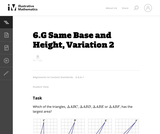Unrestricted Use
CC BY
Rating
0.0 stars

This is the second version of a task asking students to find the areas of triangles that have the same base and height. This presentation is more abstract as students are not using physical models.

Subject:
Mathematics
Geometry
Material Type:
Activity/Lab
Provider:
Illustrative Mathematics
Provider Set:
Illustrative Mathematics
Author:
Illustrative Mathematics
05/01/2012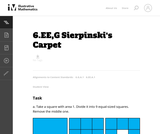Unrestricted Use
CC BY
Rating
0.0 stars

This is a task from the Illustrative Mathematics website that is one part of a complete illustration of the standard to which it is aligned. Each task has at least one solution and some commentary that addresses important asects of the task and its potential use. Here are the first few lines of the commentary for this task: Take a square with area 1. Divide it into 9 equal-sized squares. Remove the middle one. What is the area of the figure now? Take the remaining 8 square...

Subject:
Mathematics
Material Type:
Activity/Lab
Provider:
Illustrative Mathematics
Provider Set:
Illustrative Mathematics
Author:
Illustrative Mathematics
08/06/2013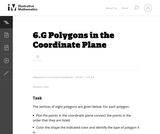Unrestricted Use
CC BY
Rating
0.0 stars

This is a task from the Illustrative Mathematics website that is one part of a complete illustration of the standard to which it is aligned. Each task has at least one solution and some commentary that addresses important asects of the task and its potential use. Here are the first few lines of the commentary for this task: The vertices of eight polygons are given below. For each polygon: * Plot the points in the coordinate plane connect the points in the order that they a...

Subject:
Mathematics
Material Type:
Activity/Lab
Provider:
Illustrative Mathematics
Provider Set:
Illustrative Mathematics
Author:
Illustrative Mathematics
09/08/2013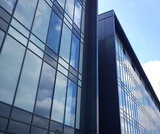Conditional Remix & Share Permitted
CC BY-NC
Rating
0.0 stars

Lesson OverviewStudents find the area of a parallelogram by rearranging it to form a rectangle. They find the area of a trapezoid by putting together two copies of it to form a parallelogram. By doing these activities and by analyzing the dimensions and areas of several examples of each figure, students develop and understand area formulas for parallelograms and trapezoids.Key ConceptsA parallelogram is a quadrilateral with two pairs of parallel sides. The base of a parallelogram can be any of the four sides. The height is the perpendicular distance from the base to the opposite side.A trapezoid is a quadrilateral with exactly one pair of parallel sides. The bases of a trapezoid are the parallel sides. The height is the perpendicular distance between the bases.You can cut a parallelogram into two pieces and reassemble them to form a rectangle. Because the area does not change, the area of the rectangle is the same as the area of the parallelogram. This gives the parallelogram area formula A = bh.You can put two identical trapezoids together to form a parallelogram with the same height as the trapezoid and a base length equal to the sum of the base lengths of the trapezoid. The area of the parallelogram is (b1 + b2)h, so the area of the trapezoid is one-half of this area. Thus, the trapezoid area formula is A = 12(b1 + b2)h.Goals and Learning ObjectivesDevelop and explore the formula for the area of a parallelogram.Develop and explore the formula for the area of a trapezoid.

Subject:
Geometry
Material Type:
Lesson Plan
Provider:
Pearson